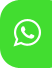## Product Detail## Ignou solved assignment for EEC-13

University  IGNOU
Service Type Solved Assignment
Course BA(Economics)
Semester
Short Name or Subject Code EEC-13
Product BA(Economics) of Solved Assignment (IGNOU)
Pattern
Price
Click to view price

### TECHNIQUES

Programme Code: BDP

Course Code: EEC-13

Assignment Code: EEC-14/AST/TMA/2019-20

Maximum Marks: 100

A. Long Answer Questions 2 x 20 = 40 marks

1.  (a) Distinguish between census and sample survey.

(b) What are the advantages and disadvantages of sample survey over the census?

(c) Prepare a short questionnaire for the collection of household data on consumption expenditure.

2.  (a) What is a standard normal variable? What are its properties?

(b) Find out the area under the standard normal curve for each of the following (use stable).

Sketch each one of them.

(i) between z = 0 and z = 0.78

(ii) between z = – 0.56 and z = 0

(iii) between z = – 0.43 and z = 0.78

(iv) between z = 0.44 and z = 1.50

(v) to the right of z = – 1.33.

B. Medium Answer Questions                                                                                              4 x 12= 48 marks

3) Explain the concepts of Skewness and Kurtosis. Briefly describe how these can be measured.

4) Bring out the salient features of the binomial distribution. 4

5)  (a) Distinguish between the concepts of correlation and regression.

(b) Find out the regression coefficients for the model 𝑌 = 𝑎 + 𝑏𝑋 on the basis of the following data

. X 1 2 3 4 5 6 7 9 11 12

Y 17 27 28 30 25 35 26 30 35 37

6) What is an index number? What are the properties that a good index number should fulfil?

C. Short Answer Questions                                                                                           2 x 6= 12 marks

7) Write short notes on the following:

(a) Standard error

(b) Life Table

8) Distinguish between stratified random sampling and systematic random sampling.